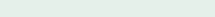# Math Puzzles Puzzle 52 Answer AndroidMath puzzle game level 52 solution with detailed hints and explanation, Scroll below to find out.

Math puzzles increase your logical thinking. The objective of the game is to find patterns hidden in the puzzle in order to arrive at the solution. Challenge yourself with different levels of math puzzles and stretch the limits of your intelligence.

### Math Puzzles Puzzle 52:

How many triangles in are there ⭐Solution/Hint:

12 triangles are small triangles

6 triangles are medium triangles

2 big triangles

If there is any Doubt or you are unable to understand the solution, then please let me know in comments, I will surely help you.

### 2 thoughts on “Math Puzzles Game Level 52 Answer with Solution”

•January 26, 2019 at 23:59
•